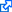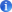# Τίτλοι που παρέχονται μέσω της Βι.Κε.Π.

Εμφάνιση περιοδικών θεματικής περιοχής: Mathematics

ΤίτλοςΘεματικές Ενότητες
Annals of probability - TheMathematics | Probabilities | Science | Statistics |
Annals of statistics - TheMathematical statistics | Mathematics | Science |
Conformal geometry and dynamicsMathematics | Conformal geometry | Dynamics |
International journal of bifurcation and chaos in applied sciences and engineeringMathematics | Computer Science | Dynamics | Cellular automata | Computational complexity |
Journal of applied probabilityMathematics | Mathematical statistics | Mathematical models | Probabilities |
Journal of the American statistical associationMathematics | Statistics |
Mathematical modelling of natural phenomenaMathematical models | Mathematics | Simulation methods |
Mathematics of computationComputer Science | Mathematics |
Representation theoryMathematics | Algebra | Group theory | Representation of groups |
Theory of probability and its applicationsMathematics | Probabilities |
Δελτίο της Ελληνικής Μαθηματικής ΕταιρίαςMathematics |
Μαθηματική ΕπιθεώρησηMathematics |